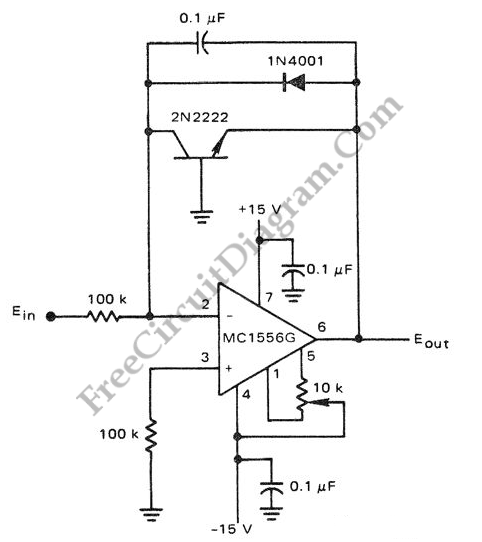# Logarithmic Amplifier Using MC1556 Op-AmpThe circuit below is a logarithmic amplifier using an MC1556 op amp. The 10K pot is an offset adjustment.  Here is  a schematic diagram of one classic example of  general purpose  logarithmic converter circuit employing LM1556 op-amp:The popularity of logarithmic amplifier is decreasing as the microprocessor and microcontroller chips are  getting lower and lower in price. Long time ago, logarithmic processing in analog domain help solving many analog computation problem, like converting division operation into simple addition. Is logarithmic conversion circuit still find a suitable application these days? Fortunately yes, although only in limited areas.  Analog logarithmic processing is still useful in pre-processing the signal before digitization, or sometimes when we need logarithmic scaling for analog display. [Circuit’s schematic diagram source: Motorola Semiconductor Product]